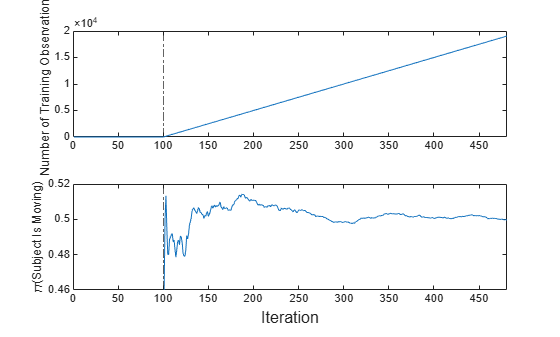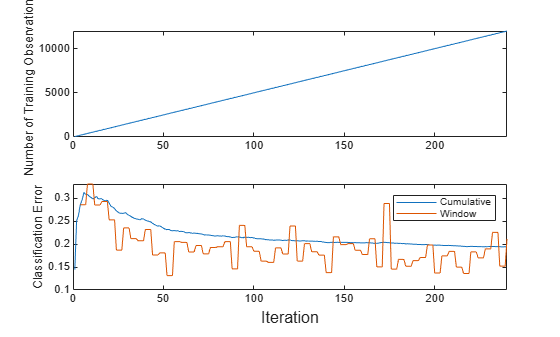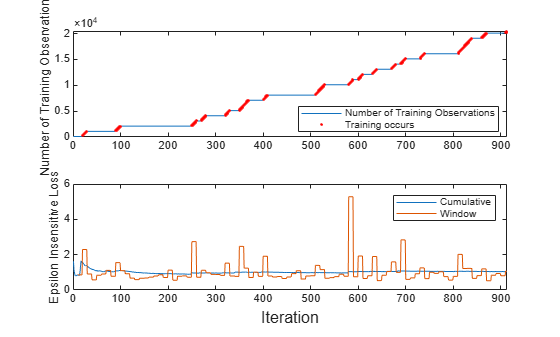# fit

Train kernel model for incremental learning

## Syntax

Mdl = fit(Mdl,X,Y)
Mdl = fit(Mdl,X,Y,Weights=weights)

## Description

The fit function fits a configured incremental learning model for kernel regression (incrementalRegressionKernel object) or binary kernel classification (incrementalClassificationKernel object) to streaming data. To additionally track performance metrics using the data as it arrives, use updateMetricsAndFit instead.

To fit or cross-validate a kernel regression or classification model to an entire batch of data at once, see fitrkernel or fitckernel, respectively.

example

Mdl = fit(Mdl,X,Y) returns an incremental learning model Mdl, which represents the input incremental learning model Mdl trained using the predictor and response data, X and Y respectively. Specifically, fit implements the following procedure: Initialize the solver with the model parameters and configurations of the input incremental learning model Mdl.Fit the model to the data, and store the updated model parameters and configurations in the output model Mdl. The input and output models have the same data type.

example

Mdl = fit(Mdl,X,Y,Weights=weights) also sets observation weights.

## Examples

collapse all

Configure incremental learning options for an incrementalClassificationKernel model object when you call the incrementalClassificationKernel function. Fit the model to incoming observations.

Create an incremental kernel model for binary classification. Specify an estimation period of 5000 observations and the stochastic gradient descent (SGD) solver.

Mdl = incrementalClassificationKernel(EstimationPeriod=5000,Solver="sgd")
Mdl = incrementalClassificationKernel IsWarm: 0 Metrics: [1x2 table] ClassNames: [1x0 double] ScoreTransform: 'none' NumExpansionDimensions: 0 KernelScale: 1 Properties, Methods 

Mdl is an incrementalClassificationKernel model. All its properties are read-only.

Mdl must be fit to data before you can use it to perform any other operations.

Load the human activity data set. Randomly shuffle the data.

load humanactivity n = numel(actid); rng(1) % For reproducibility idx = randsample(n,n); X = feat(idx,:); Y = actid(idx);

For details on the data set, enter Description at the command line.

Responses can be one of five classes: Sitting, Standing, Walking, Running, or Dancing. Dichotomize the response by identifying whether the subject is moving (actid > 2).

Y = Y > 2;

Fit the incremental model to the training data, in chunks of 50 observations at a time, by using the fit function. At each iteration:

• Simulate a data stream by processing 50 observations.

• Overwrite the previous incremental model with a new one fitted to the incoming observations.

• Store the number of training observations and the prior probability of whether the subject moved (Y = true) to see how they evolve during incremental training.

% Preallocation numObsPerChunk = 50; nchunk = floor(n/numObsPerChunk); numtrainobs = zeros(nchunk,1); priormoved = zeros(nchunk,1); % Incremental fitting for j = 1:nchunk ibegin = min(n,numObsPerChunk*(j-1) + 1); iend = min(n,numObsPerChunk*j); idx = ibegin:iend; Mdl = fit(Mdl,X(idx,:),Y(idx)); numtrainobs(j) = Mdl.NumTrainingObservations; priormoved(j) = Mdl.Prior(Mdl.ClassNames == true); end

Mdl is an incrementalClassificationKernel model object trained on all the data in the stream.

To see how the parameters evolve during incremental learning, plot them on separate tiles.

t = tiledlayout(2,1); nexttile plot(numtrainobs) xlim([0 nchunk]) ylabel("Number of Training Observations") xline(Mdl.EstimationPeriod/numObsPerChunk,"-.") nexttile plot(priormoved) xlim([0 nchunk]) ylabel("\pi(Subject Is Moving)") xline(Mdl.EstimationPeriod/numObsPerChunk,"-.") xlabel(t,"Iteration")The plot suggests that fit does not fit the model to the data or update the parameters until after the estimation period.

Train a kernel model for binary classification by using fitckernel, and convert it to an incremental learner by using incrementalLearner. Track the model performance and fit the model to streaming data. Specify the observation weights when you call fitckernel and incremental learning functions.

Load the human activity data set. Randomly shuffle the data.

load humanactivity rng(1) % For reproducibility n = numel(actid); idx = randsample(n,n); X = feat(idx,:); Y = actid(idx);

For details on the data set, enter Description at the command line.

Responses can be one of five classes: Sitting, Standing, Walking, Running, or Dancing. Dichotomize the response by identifying whether the subject is moving (actid > 2).

Y = Y > 2;

Suppose that the data collected when the subject was not moving (Y = false) has double the quality than when the subject was moving. Create a weight variable that attributes 2 to observations collected from a stationary subject, and 1 to a moving subject.

W = ones(n,1) + ~Y;

Train Kernel Model for Binary Classification

Fit a kernel model for binary classification to a random sample of half the data.

idxtt = randsample([true false],n,true); TTMdl = fitckernel(X(idxtt,:),Y(idxtt),Weights=W(idxtt))
TTMdl = ClassificationKernel ResponseName: 'Y' ClassNames: [0 1] Learner: 'svm' NumExpansionDimensions: 2048 KernelScale: 1 Lambda: 8.2967e-05 BoxConstraint: 1 Properties, Methods 

TTMdl is a ClassificationKernel model object representing a traditionally trained kernel model for binary classification.

Convert Trained Model

Convert the traditionally trained classification model to a model for incremental learning.

IncrementalMdl = incrementalLearner(TTMdl)
IncrementalMdl = incrementalClassificationKernel IsWarm: 1 Metrics: [1x2 table] ClassNames: [0 1] ScoreTransform: 'none' NumExpansionDimensions: 2048 KernelScale: 1 Properties, Methods 

IncrementalMdl is an incrementalClassificationKernel model. All its properties are read-only.

Separately Track Performance Metrics and Fit Model

Perform incremental learning on the rest of the data by using the updateMetrics and fit functions. At each iteration:

1. Simulate a data stream by processing 50 observations at a time.

2. Call updateMetrics to update the cumulative and window classification error of the model given the incoming chunk of observations. Overwrite the previous incremental model to update the losses in the Metrics property. Note that the function does not fit the model to the chunk of data—the chunk is "new" data for the model. Specify the observation weights.

3. Call fit to fit the model to the incoming chunk of observations. Overwrite the previous incremental model to update the model parameters. Specify the observation weights.

4. Store the classification error and number of training observations.

% Preallocation idxil = ~idxtt; nil = sum(idxil); numObsPerChunk = 50; nchunk = floor(nil/numObsPerChunk); ce = array2table(zeros(nchunk,2),VariableNames=["Cumulative","Window"]); numtrainobs = [zeros(nchunk,1)]; Xil = X(idxil,:); Yil = Y(idxil); Wil = W(idxil); % Incremental fitting for j = 1:nchunk ibegin = min(nil,numObsPerChunk*(j-1) + 1); iend = min(nil,numObsPerChunk*j); idx = ibegin:iend; IncrementalMdl = updateMetrics(IncrementalMdl,Xil(idx,:),Yil(idx), ... Weights=Wil(idx)); ce{j,:} = IncrementalMdl.Metrics{"ClassificationError",:}; IncrementalMdl = fit(IncrementalMdl,Xil(idx,:),Yil(idx), ... Weights=Wil(idx)); numtrainobs(j) = IncrementalMdl.NumTrainingObservations; end

IncrementalMdl is an incrementalClassificationKernel model object trained on all the data in the stream.

Alternatively, you can use updateMetricsAndFit to update performance metrics of the model given a new chunk of data, and then fit the model to the data.

Plot a trace plot of the number of training observations and the performance metrics.

t = tiledlayout(2,1); nexttile plot(numtrainobs) xlim([0 nchunk]) ylabel("Number of Training Observations") nexttile plot(ce.Variables) xlim([0 nchunk]) legend(ce.Properties.VariableNames) ylabel("Classification Error") xlabel(t,"Iteration")The plot suggests that the fit function fits the model during all incremental learning iterations. The cumulative loss is stable and gradually decreases, whereas the window loss jumps.

Incrementally train a kernel regression model only when its performance degrades.

Load and shuffle the 2015 NYC housing data set. For more details on the data, see NYC Open Data.

load NYCHousing2015 rng(1) % For reproducibility n = size(NYCHousing2015,1); shuffidx = randsample(n,n); NYCHousing2015 = NYCHousing2015(shuffidx,:);

Extract the response variable SALEPRICE from the table. For numerical stability, scale SALEPRICE by 1e6.

Y = NYCHousing2015.SALEPRICE/1e6; NYCHousing2015.SALEPRICE = [];

To reduce computational cost for this example, remove the NEIGHBORHOOD column, which contains a categorical variable with 254 categories.

NYCHousing2015.NEIGHBORHOOD = [];

Create dummy variable matrices from the other categorical predictors.

catvars = ["BOROUGH","BUILDINGCLASSCATEGORY"]; dumvarstbl = varfun(@(x)dummyvar(categorical(x)),NYCHousing2015, ... InputVariables=catvars); dumvarmat = table2array(dumvarstbl); NYCHousing2015(:,catvars) = [];

Treat all other numeric variables in the table as predictors of sales price. Concatenate the matrix of dummy variables to the rest of the predictor data.

idxnum = varfun(@isnumeric,NYCHousing2015,OutputFormat="uniform"); X = [dumvarmat NYCHousing2015{:,idxnum}];

Configure a kernel regression model for incremental learning so that it does not have an estimation or metrics warm-up period. Specify a metrics window size of 1000. Prepare the model for updateMetrics by fitting it to the first 100 observations.

Mdl = incrementalRegressionKernel(EstimationPeriod=0, ... MetricsWarmupPeriod=0,MetricsWindowSize=1000); initobs = 100; Mdl = fit(Mdl,X(1:initobs,:),Y(1:initobs));

Mdl is an incrementalRegressionKernel model object.

Perform incremental learning, with conditional fitting, by following this procedure for each iteration:

• Simulate a data stream by processing a chunk of 100 observations at a time.

• Update the model performance by computing the epsilon insensitive loss, within a 200 observation window.

• Fit the model to the chunk of data only when the loss more than doubles from the minimum loss experienced.

• When tracking performance and fitting, overwrite the previous incremental model.

• Store the epsilon insensitive loss and number of training observations to see how they evolve during training.

• Track when fit trains the model.

% Preallocation numObsPerChunk = 100; nchunk = floor((n - initobs)/numObsPerChunk); ei = array2table(nan(nchunk,2),VariableNames=["Cumulative","Window"]); numtrainobs = zeros(nchunk,1); trained = false(nchunk,1); % Incremental fitting for j = 1:nchunk ibegin = min(n,numObsPerChunk*(j-1) + 1 + initobs); iend = min(n,numObsPerChunk*j + initobs); idx = ibegin:iend; Mdl = updateMetrics(Mdl,X(idx,:),Y(idx)); ei{j,:} = Mdl.Metrics{"EpsilonInsensitiveLoss",:}; minei = min(ei{:,2}); pdiffloss = (ei{j,2} - minei)/minei*100; if pdiffloss > 100 Mdl = fit(Mdl,X(idx,:),Y(idx)); trained(j) = true; end numtrainobs(j) = Mdl.NumTrainingObservations; end

Mdl is an incrementalRegressionKernel model object trained on all the data in the stream.

To see how the number of training observations and model performance evolve during training, plot them on separate tiles.

t = tiledlayout(2,1); nexttile plot(numtrainobs) hold on plot(find(trained),numtrainobs(trained),"r.") xlim([0 nchunk]) ylabel("Number of Training Observations") legend("Number of Training Observations","Training occurs",Location="best") hold off nexttile plot(ei.Variables) xlim([0 nchunk]) ylabel("Epsilon Insensitive Loss") legend(ei.Properties.VariableNames) xlabel(t,"Iteration")The trace plot of the number of training observations shows periods of constant values, during which the loss does not double from the minimum experienced.

## Input Arguments

collapse all

Incremental learning model to fit to streaming data, specified as an incrementalClassificationKernel or incrementalRegressionKernel model object. You can create Mdl directly or by converting a supported, traditionally trained machine learning model using the incrementalLearner function. For more details, see the corresponding reference page.

Chunk of predictor data, specified as a floating-point matrix of n observations and Mdl.NumPredictors predictor variables.

The length of the observation labels Y and the number of observations in X must be equal; Y(j) is the label of observation j (row) in X.

Note

• If Mdl.NumPredictors = 0, fit infers the number of predictors from X, and sets the corresponding property of the output model. Otherwise, if the number of predictor variables in the streaming data changes from Mdl.NumPredictors, fit issues an error.

• fit supports only floating-point input predictor data. If your input data includes categorical data, you must prepare an encoded version of the categorical data. Use dummyvar to convert each categorical variable to a numeric matrix of dummy variables. Then, concatenate all dummy variable matrices and any other numeric predictors. For more details, see Dummy Variables.

Data Types: single | double

Chunk of responses (labels), specified as a categorical, character, or string array, a logical or floating-point vector, or a cell array of character vectors for classification problems; or a floating-point vector for regression problems.

The length of the observation labels Y and the number of observations in X must be equal; Y(j) is the label of observation j (row) in X.

For classification problems:

• fit supports binary classification only.

• When the ClassNames property of the input model Mdl is nonempty, the following conditions apply:

• If Y contains a label that is not a member of Mdl.ClassNames, fit issues an error.

• The data type of Y and Mdl.ClassNames must be the same.

Data Types: char | string | cell | categorical | logical | single | double

Chunk of observation weights, specified as a floating-point vector of positive values. fit weighs the observations in X with the corresponding values in weights. The size of weights must equal n, the number of observations in X.

By default, weights is ones(n,1).

For more details, including normalization schemes, see Observation Weights.

Data Types: double | single

Note

• If an observation (predictor or label) or weight contains at least one missing (NaN) value, fit ignores the observation. Consequently, fit uses fewer than n observations to create an updated model, where n is the number of observations in X.

• The chunk size n and the stochastic gradient descent (SGD) hyperparameter mini-batch size (Mdl.SolverOptions.BatchSize) can be different values, and n does not have to be an exact multiple of the mini-batch size. fit uses the BatchSize observations when it applies SGD for each learning cycle. The number of observations in the last mini-batch for the last learning cycle can be less than or equal to Mdl.SolverOptions.BatchSize.

## Output Arguments

collapse all

Updated incremental learning model, returned as an incremental learning model object of the same data type as the input model Mdl, either incrementalClassificationKernel or incrementalRegressionKernel.

If Mdl.EstimationPeriod > 0, fit estimates hyperparameters using the first Mdl.EstimationPeriod observations passed to it; the function does not train the input model using that data. However, if an incoming chunk of n observations is greater than or equal to the number of observations remaining in the estimation period m, fit estimates hyperparameters using the first nm observations, and fits the input model to the remaining m observations. Consequently, the software updates model parameters, hyperparameter properties, and recordkeeping properties such as NumTrainingObservations.

For classification problems, if the ClassNames property of the input model Mdl is an empty array, fit sets the ClassNames property of the output model Mdl to unique(Y).

## Tips

• Unlike traditional training, incremental learning might not have a separate test (holdout) set. Therefore, to treat each incoming chunk of data as a test set, pass the incremental model and each incoming chunk to updateMetrics before training the model on the same data.

## Algorithms

collapse all

### Observation Weights

For classification problems, if the prior class probability distribution is known (in other words, the prior distribution is not empirical), fit normalizes observation weights to sum to the prior class probabilities in the respective classes. This action implies that observation weights are the respective prior class probabilities by default.

For regression problems or if the prior class probability distribution is empirical, the software normalizes the specified observation weights to sum to 1 each time you call fit.

## Version History

Introduced in R2022a